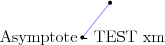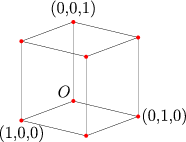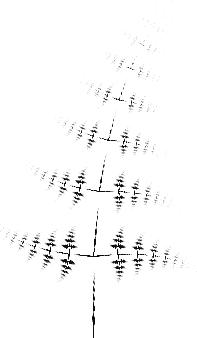# User talk:WikiSysop

(diff) ← Older revision | Latest revision (diff) | Newer revision → (diff)

Test Asymptote:

## Test Asymptote## Test Cite Extension

Example: Cite-Extension

## Test MathJax

\begin{align} \dot{x} & = \sigma(y-x) \\ \dot{y} & = \rho x - y - xz \\ \dot{z} & = -\beta z + xy \end{align}

$\frac{1}{(\sqrt{\phi \sqrt{5}}-\phi) e^{\frac25 \pi}} = 1+\frac{e^{-2\pi}} {1+\frac{e^{-4\pi}} {1+\frac{e^{-6\pi}} {1+\frac{e^{-8\pi}} {1+\ldots} } } }$

How to Cite This Entry:
WikiSysop. Encyclopedia of Mathematics. URL: http://www.encyclopediaofmath.org/index.php?title=WikiSysop&oldid=26978

Test January 12th 2015